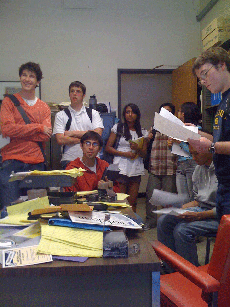## Mathematics 110---Linear Algebra

### Arthur Ogus

Office Hours:In concrete terms, linear algebra is the study of systems of linear equations in several variables. Such systems arise, often in disguise, in a vast number of situations in mathematics and other fields, and it is absolutely crucial to understand them as thoroughly and conceptually as possible. The solution set to such a system has a rich geometric structure which is a fundamental part of linear algebra---in fact I think this course could just as well be called ``Linear Geometry." The algebra and geometry are unified by the concepts of vector and inner product spaces, and a key theme of the course will be the interplay between the "abstract" linear algebra and the geometric intuition that it expresses. In the end (and in fact even in the beginning) this theme will be more important to us than the algorithmic methods for solving equations covered in math 54. An important goal of the course is for students to learn to read and write mathematical proofs, as well as to become comfortable with the interplay between abstraction and intuition. Our textbook will be:

#### Linear Algebra Done Right, by Sheldon Axler

There will be weekly assigments, due each Wednesday in your section, one or two midterms, as well as some unspecified number of quizzes given at unspecified intervals. The text this year is very succinct but readable, and I urge you to read ahead of he lectures. I will try to follow the syllabus linked to below, which will however be subject to adjustment as the course proceeds.

The grading will be approximately weighted as follows: 25% homework, 30% midterms, 40% final, 5% quizzes. I try to assign grades as follows:

1. A thorough understanding of the material, as well as a demonstration of originality in solving problems and writing proofs.
2. Very good understanding of most of the material, demonstrated by familiarity with definitions and proofs and ability to solve problems.
3. Firm grasp of the main points, including the important definitions and theorems, ability to solve standard problems.
4. Familiarility with major concepts, terminology, and problem solving technqiues.
5. None of the above.

We are in Final Exam Group 2.

For information on when and how to reach me, see my home page.

You can check my calendar here.